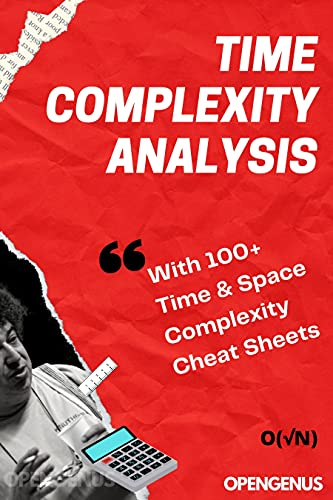×

Search anything:

# "Time Complexity Analysis" book

#### CultureFREE BOOK -> Problems for the day before your Coding Interview (on Amazon)

"Time Complexity Analysis" is a book focused on Mathematical Analysis of Time and Space Complexity of various algorithms and data structures along with basics of Time Complexity like Big-O notations.Get his book now:

This book “Time Complexity Analysis” 💻 introduces you to the basics of Time Complexity notations, meaning of the Complexity values and How to analyze various Algorithmic problems. This book includes Time and Space Complexity cheat sheets at the end as a bonus resource.

We have tackled several significant problems and demonstrated the approach to analyze them and arrived at the Time and Space Complexity of the problems and Algorithms.

This is a MUST-READ book for all Computer Science students and Programmers. Do not miss this opportunity.

You will get a better idea to judge which approach will work better and will be able to make better judgements in your development work.

See the “Table of content” to get the list of exciting topics you will learn about.

Some of the key points you will understand:

• Random Access Memory does not take O(1) time. It is complicated and in general, has a Time Complexity of O(√N).

• Multiplication takes O(N^2) time, but the most optimal Algorithm (developed in 2019) takes O(N logN) time which is believed to be the theoretical limit.

• As per Time Complexity, finding the largest element and the ith largest element takes the same order of time.

It is recommended that you go through this book twice. First time, you may skip the minute details that you may not understand at first go and get the overview.
In the second reading, you will get all the ideas, and this will strengthen your insights.

In 1950s, Computing was not a Science.

It was a collective effort by several Computer Scientists such as Robert Tarjan and Philippe Flajolet who analyzed several computational problems to demonstrate that Computation Problems are equally complicated as Physics and Mathematics Problems. The ideas captured in this book include some of these analyses which glorified Computer Science and made it a Scientific field.

Table of content:

1. Introduction to Time and Space Complexity (+ different notations)
2. How to calculate Time Complexity?
3. Meaning of different Time Complexity
4. Brief Background on NP and P
5. Does O(1) time exist?: Cost of accessing Memory
6. Time Complexity of Basic Arithmetic Operations
6.1. Bitwise operations
6.3. Subtraction
6.4. Multiplication
6.5. Division
7. Analysis of Array
8. Analysis of Dynamic Array
9. Find largest element
10. Find Second largest element
11. Find i-th largest element
12. Time Complexity Bound for comparison-based sorting
12.1. Analysis of Selection Sort
12.2. Analysis of Insertion Sort
12.3. Analysis of Bubble Sort
12.4. Analysis of Quick Sort
13. Bound for non-comparison-based sorting
13.1. Analysis of Counting Sort
13.2. Analysis of Bucket Sort
15. Analysis of Hash functions
16. Analysis of Binary Search
17. Time and Space Complexity Cheat Sheets

There is no other book that cover these topics. Many students have several misconceptions which are resolved with the book.

Book: Time Complexity Analysis
Authors: Aditya Chatterjee; Ue Kiao, PhD.
Contributors (7): Vansh Pratap Singh, Shreya Shah, Vikram Shishupalsingh Bais, Mallika Dey, Siddhant Rao, Shweta Bhardwaj, K. Sai Drishya.

Read this book and level up 🔥🔥#### Ue Kiao, PhD

Ue Kiao is a Technical Author and Software Developer with B. Sc in Computer Science at National Taiwan University and PhD in Algorithms at Tokyo Institute of Technology | Researcher at TaoBao

"Time Complexity Analysis" book